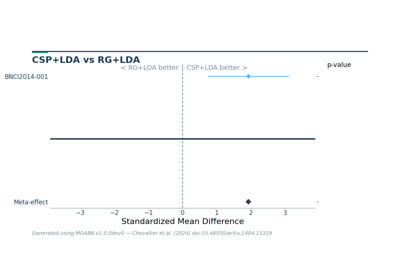# moabb.analysis.plotting.meta_analysis_plot¶

`moabb.analysis.plotting.``meta_analysis_plot`(stats_df, alg1, alg2)[source][source]

Meta-analysis to compare two algorithms across several datasets

A meta-analysis style plot that shows the standardized effect with confidence intervals over all datasets for two algorithms. Hypothesis is that alg1 is larger than alg2

Parameters
• stats_df (DataFrame) – DataFrame generated by compute_dataset_statistics

• alg1 (str) – Name of first pipeline

• alg2 (str) – Name of second pipeline

Returns

fig – Pyplot handle

Return type

Figure

## Examples using `moabb.analysis.plotting.meta_analysis_plot`¶